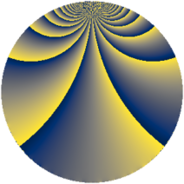# Properties

 Label 345.2.mLevel $345$ Weight $2$ Character orbit 345.m Rep. character $\chi_{345}(16,\cdot)$ Character field $\Q(\zeta_{11})$ Dimension $160$ Newform subspaces $4$ Sturm bound $96$ Trace bound $2$

# Related objects

## Defining parameters

 Level: $$N$$ $$=$$ $$345 = 3 \cdot 5 \cdot 23$$ Weight: $$k$$ $$=$$ $$2$$ Character orbit: $$[\chi]$$ $$=$$ 345.m (of order $$11$$ and degree $$10$$) Character conductor: $$\operatorname{cond}(\chi)$$ $$=$$ $$23$$ Character field: $$\Q(\zeta_{11})$$ Newform subspaces: $$4$$ Sturm bound: $$96$$ Trace bound: $$2$$ Distinguishing $$T_p$$: $$2$$

## Dimensions

The following table gives the dimensions of various subspaces of $$M_{2}(345, [\chi])$$.

Total New Old
Modular forms 520 160 360
Cusp forms 440 160 280
Eisenstein series 80 0 80

## Trace form

 $$160 q + 8 q^{2} - 4 q^{4} + 4 q^{6} + 8 q^{7} + 24 q^{8} - 16 q^{9} + O(q^{10})$$ $$160 q + 8 q^{2} - 4 q^{4} + 4 q^{6} + 8 q^{7} + 24 q^{8} - 16 q^{9} + 8 q^{10} + 32 q^{11} + 8 q^{13} + 16 q^{14} + 4 q^{15} - 68 q^{16} - 28 q^{17} + 8 q^{18} - 20 q^{19} - 64 q^{22} + 24 q^{23} + 12 q^{24} - 16 q^{25} - 24 q^{26} + 64 q^{28} + 4 q^{30} + 8 q^{32} + 8 q^{33} + 10 q^{34} - 32 q^{35} - 48 q^{36} + 64 q^{37} - 148 q^{38} - 36 q^{39} + 2 q^{40} - 36 q^{41} + 24 q^{42} - 40 q^{43} + 60 q^{44} - 104 q^{46} + 56 q^{47} - 144 q^{48} + 36 q^{49} - 36 q^{50} - 56 q^{51} + 64 q^{52} - 32 q^{53} - 18 q^{54} - 28 q^{55} - 132 q^{56} + 8 q^{57} - 20 q^{58} + 16 q^{59} - 10 q^{60} + 4 q^{61} + 64 q^{62} + 8 q^{63} + 28 q^{64} + 32 q^{65} + 56 q^{66} + 20 q^{67} + 152 q^{68} + 24 q^{69} + 100 q^{71} + 24 q^{72} + 28 q^{73} + 60 q^{74} + 56 q^{76} - 120 q^{77} + 16 q^{78} - 132 q^{79} + 32 q^{80} - 16 q^{81} - 184 q^{82} - 112 q^{83} + 48 q^{84} + 20 q^{85} + 136 q^{86} + 8 q^{87} - 144 q^{88} - 160 q^{89} + 8 q^{90} + 80 q^{91} - 228 q^{92} + 16 q^{93} - 220 q^{94} + 16 q^{95} - 16 q^{96} - 168 q^{97} - 144 q^{98} + 32 q^{99} + O(q^{100})$$

## Decomposition of $$S_{2}^{\mathrm{new}}(345, [\chi])$$ into newform subspaces

Label Dim $A$ Field CM Traces $q$-expansion
$a_{2}$ $a_{3}$ $a_{5}$ $a_{7}$
345.2.m.a $30$ $2.755$ None $$0$$ $$3$$ $$3$$ $$1$$
345.2.m.b $30$ $2.755$ None $$6$$ $$-3$$ $$-3$$ $$7$$
345.2.m.c $50$ $2.755$ None $$0$$ $$-5$$ $$5$$ $$-3$$
345.2.m.d $50$ $2.755$ None $$2$$ $$5$$ $$-5$$ $$3$$

## Decomposition of $$S_{2}^{\mathrm{old}}(345, [\chi])$$ into lower level spaces

$$S_{2}^{\mathrm{old}}(345, [\chi]) \cong$$ $$S_{2}^{\mathrm{new}}(23, [\chi])$$$$^{\oplus 4}$$$$\oplus$$$$S_{2}^{\mathrm{new}}(69, [\chi])$$$$^{\oplus 2}$$$$\oplus$$$$S_{2}^{\mathrm{new}}(115, [\chi])$$$$^{\oplus 2}$$